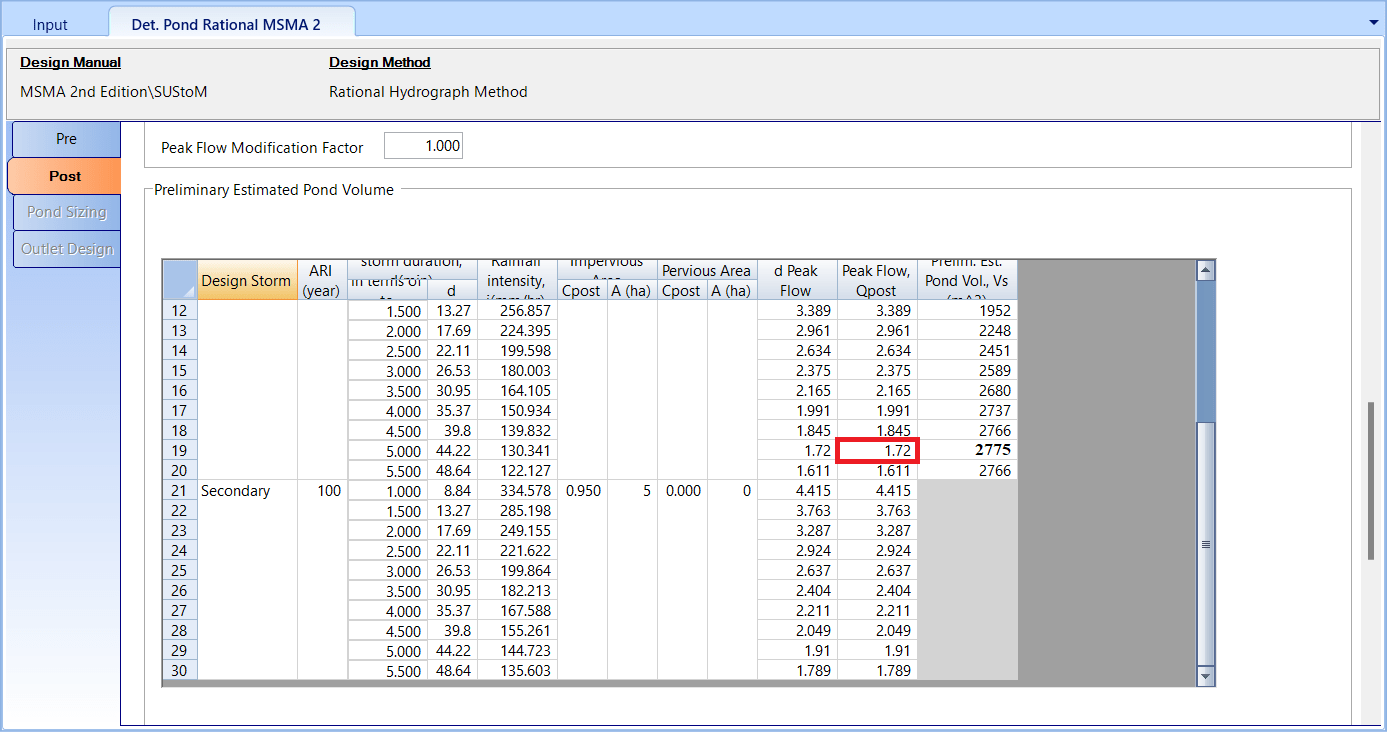603 - 5885 1250 (4 lines) info@mes100.com
Select Page

# Case Study on the Differences of Pond and OSD Swinburne Method

A case study between OSD Swinburne Method and Pond Swinburne Method has been done, to compare the difference between these two stormwater managements.

Project references:

Project File for Pond Swinburne Method

Project File for OSD Swinburne Method

# Similarity between two Swinburne methods#

Swinburne method is comparing between the discharge of Pre development and Post Development, in order to determine the dimension of the control (OSD/Pond) and the size of outlets.

# The applicability of the two Swinburne methods#

OSD is suitable to be used for a development area of less than 5 ha, while Pond is used for an area larger than 5 ha. However, MSMA 2nd Edition has come out with a shorter, simplified method for OSD called “Simplified Method” and is often encouraged by the authority to use this new method. (refer blog Comparison of OSD results between software calculation and excel spreadsheet using ‘Simplified Method)

# The difference in methodology: A summary#

Same concept is applied for both OSD and Pond, however there are some differences in terms of the calculation, which results to different output. Below are the examples:

i) PRE DEVELOPMENT

Given the same situation of pre development;

Location: Kuala Lumpur – Puchong Drop, K Lumpur

Time of concentration:

L (m) = 315

S (m/km) =

Area (ha) =

Tc (min) = 21.6

The ARI used for comparison is 50 years

For Pre Development Discharge (Qpre), both are calculated by using Swinburne Equation Q=CiA

Therefore, Qpre = 1.123 m3/s

The difference between OSD and Pond as you can see in the calculation is that Pond considers the calculation of three ARI which consists of Minor, Major and Secondary. In this case, the comparison is made by using Major design value.

ii) POST DEVELOPMENT

Given the same details for the Post Development;

Overland Flow Time, td

L (m) = 10

S (%) = 2

N = 0.015

To(min) = 3.01

Drain Flow Time, tc

L (m) = 350

V (m/s) = 1

Td(min) = 5.83

Therefore, tc = 8.8 min

Catchment Runoff Coefficient

Total Area = 5 ha (impervious)

C Impervious = 0.95

The calculation of Post Development Discharge (Qpost) is different between OSD and Pond. For OSD, Rational Equation Q=CiA is used while for Pond, Rational Hydrograph Method (RHM) is used to determine the Qpost.

RHM is used to route the Preliminary Pond Volume and is used to determine the Qpost which is based on the highest pond volume. Due to the difference, the end output of the Qpost also will be different.Pond: Preliminary pond volume is calculated based on RHM and the peak Qpost is determined based on the highest preliminary pond volume

The Pond Qpost is 1.72 m3/s which is based on the highest preliminary pond volume, 2775 m3.

The OSD Qpost is 3.976 m3/s.

iii) POND STORAGE

The estimation of pond sizing is based on the calculated Preliminary Pond Volume. Based on this project, the volume is 2775 m3 at 1.72 m3/s discharge.

OSD dimension estimation is based on the Site Storage Requirement (SSR), which also consider the value of tcs (refer to MSMA 1st Edition Chapter 19, Figure 19.2)

Based on the result, the SSR is 2257.92 m3 with an additional 20% to account for inaccuracies in construction and further loss of storage due to build-up of the lawn surface.

OSD SSR = 2257.92 x 1.2 = 2709.505 m3

iv) POND SIZING

The preliminary pond volume and the SSR are referred to estimate the pond volume. To determine the end dimension, the calculation of the dimension has also to be compared to the outlet design (based on trial and error)

v) OUTLET DESIGN

Pond outlet design is accounting for the minor, major and secondary design (emergency spillway). The adjustment of the orifice sizing and the number are adjusted to comply with the requirement of MSMA Qpost < Qpre.

Inflow hydrograph routing also is involved in order to calculate maximum water level.